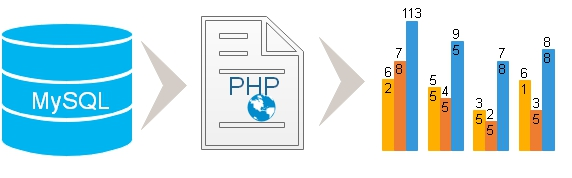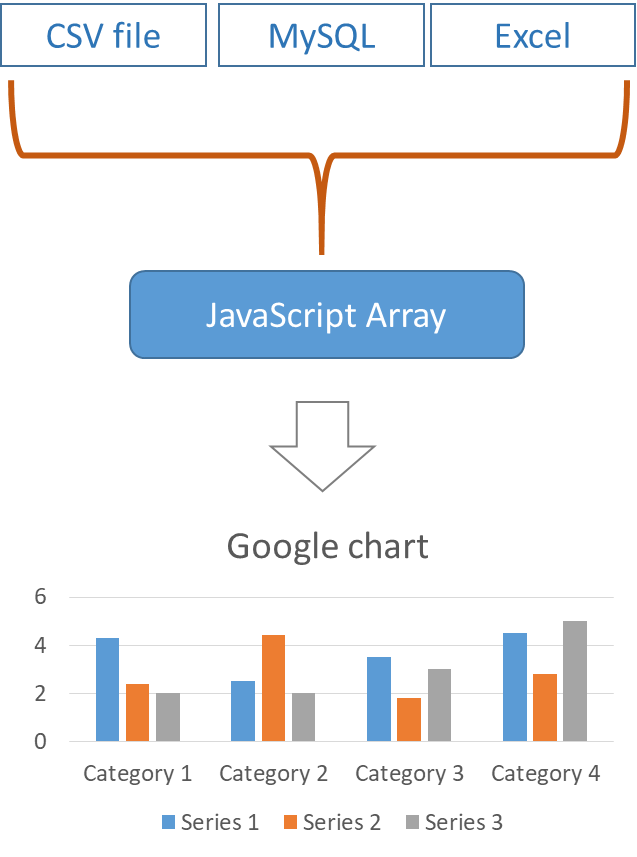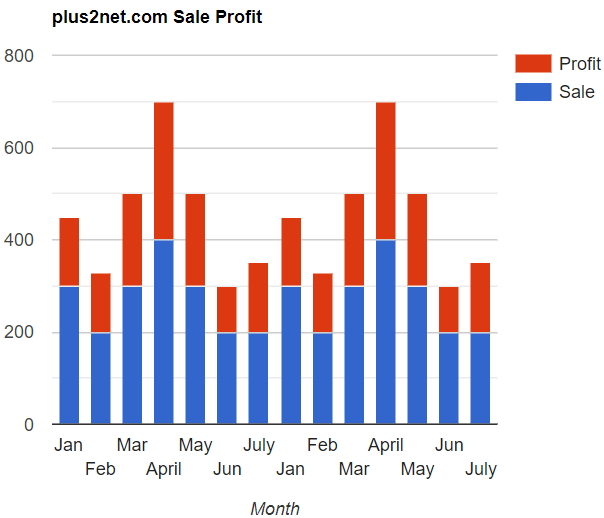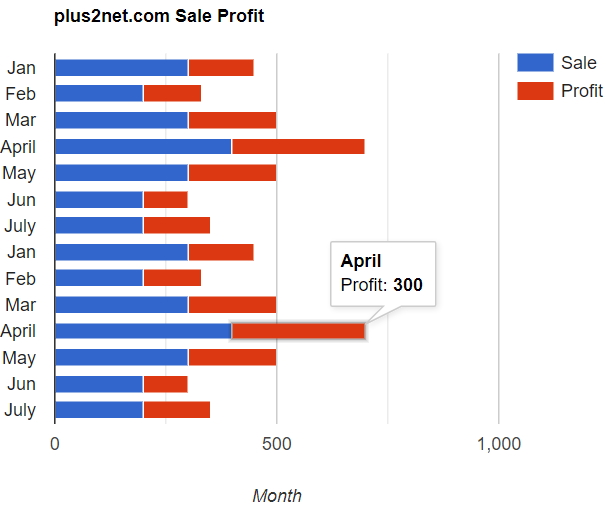# Column Chart using data from MySQL table by PHPFile NameDetails
config.php MySQLi Database connection details are stored here.
sql_dump.txt SQL Dump to create chart_data_column table with sample data.
readme.txt Instructions on how to run the script
index.php The main file to display records and the Column /bar chart.
Using PDO : PHP Data Object
File NameDetails
config-pdo.php PDO Database connection details are stored here.
index-pdo.php Using PDO the main file to display column / bar Chart.
Using CSV (Comma Separated Value) file :
File NameDetails
index-csv.php Reading CSV file by using fgetcsv() and display chart.
chart_data_column.csv Comma separated value ( csv ) file with data.

Column Chart : Orientation is vertical
Bar Chart : Orientation is horizontal

Column & Bar Chart with data from MySQL database table using PHP pdo and google chart library

## Sample column Chart in html

Without using PHP or MySQL, you can try this simple HTML and JavaScript code to display the above column chart. Copy this code and save ( open ) as HTML file. Google Chart library is also included inside the code.

## 1 : Collecting data from MySQL database

After connecting to database through config.php file, we will run the SQL to collect the data from MySQL table. You can read more on MySQLi and how to collect data from table.
``````if(\$stmt = \$connection->query("SELECT month,sale,profit FROM chart_data_column")){

echo "No of records : ".\$stmt->num_rows."<br>";
\$php_data_array = Array(); // create PHP array
echo "<table>
<tr> <th>Month</th><th>Sale</th><th>Profit</th></tr>";
while (\$row = \$stmt->fetch_row()) {
echo "<tr><td>\$row</td><td>\$row</td><td>\$row</td></tr>";
\$php_data_array[] = \$row; // Adding to array
}
echo "</table>";
}else{
echo \$connection->error;
}``````
Using PDO : PHP Data Object we can connect to MySQL and retrive the data to create the array \$php_data_array.
``````<?Php
require "config-pdo.php";// Database connection
\$query="SELECT month,sale,profit FROM chart_data_column";
\$step = \$dbo->prepare(\$query);
if(\$step->execute()){
\$php_data_array = \$step->fetchAll(); //
echo "<script>
var my_2d = ".json_encode(\$php_data_array)."
</script>";
}
?>``````

## 2 : Data array in PHP

Before displaying records in a table we have created an array in PHP ( `\$php_data_array`). While displaying the records in a table we store each record inside the PHP array.
``\$php_data_array[] = \$row; // Adding to array``
After displaying all the records in a table we have the `\$php_data_array` with all the data collected from MySQL table. We can display the Json string like this.
``echo json_encode(\$php_data_array);``

## 3 : Transferring data from PHP to JavaScript to create the chartBy using json_encode we will create an json string which can be used to create the JavaScript two dimensional array.
``````echo "<script>
var my_2d = ".json_encode(\$php_data_array)."
</script>";``````

## 4 : Adding data to Chart

Our JavaScript array `my_2d` stores all the data required for creating the chart. We need to display them in the format required by our Chart library.
``````for(i = 0; i < my_2d.length; i++)
We used parseInt() function to convert input string value to Integer. JavaScript part is here .
``````<script type="text/javascript" src="https://www.gstatic.com/charts/loader.js"></script>
<script type="text/javascript">

// Load the Visualization API and the corechart package.

function drawChart() {

// Create the data table.
for(i = 0; i < my_2d.length; i++)
var options = {
title: 'plus2net.com Sales Profit',
hAxis: {title: 'Month',  titleTextStyle: {color: '#333'}},
vAxis: {minValue: 0}
};

chart.draw(data, options);
}
///////////////////////////////
////////////////////////////////////
</script>``````

## 5. HTML part

We can show the chart inside a <DIV> tag and place the same any where we want to display the chart.
``<div id="chart_div"></div>``

The chart drawn above can be displayed with live internet connection as the library is to be loaded from gstatic.com

Data is collected from MySQL database table so data can be edited by using script and the same will be reflected by the Chart. We have not included this part in the present script and there are many tutorials available here one handling data using PHP and MySQL.

You can read tutorials on other types of charts displayed by using data from database.

## Stacked Column chart``````var options = {
title: 'plus2net.com Sale Profit',
hAxis: {title: 'Month',  titleTextStyle: {color: '#333'}},
vAxis: {minValue: 0},
height:400,
width:480,
isStacked:true
};``````

## Bar ChartChange this line
``````/*
var chart =
*/
var chart =

## Using CSV file as data source

Once the php aray `\$php_data_array` is created the rest of the code remain same. Here we are reading the chart_data_column.csv file to create the `\$php_data_array`. Once the array is created same code can be used. Zip file below contains the chart_data_column.csv file as data source and the index-csv.php file to display the chart.
We used fgetcsv() to read csv file
``````\$f_pointer=fopen("chart_data_column.csv","r"); // file pointer
\$php_data_array = Array(); // create PHP array
while(! feof(\$f_pointer)){
\$ar=fgetcsv(\$f_pointer);
//echo print_r(\$ar); // print the array
\$php_data_array[] = \$ar; // Adding to array
}
print_r(\$php_data_array);
echo "<script>
var my_2d=".json_encode(\$php_data_array)."
</script>";``````## Subscribe

* indicates required
Subscribe to plus2netplus2net.com